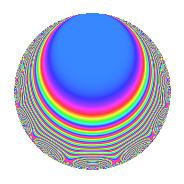# Properties

 Label 1800.1.baLevel 1800 Weight 1 Character orbit ba Rep. character $$\chi_{1800}(499,\cdot)$$ Character field $$\Q(\zeta_{6})$$ Dimension 12 Newforms 3 Sturm bound 360 Trace bound 9

# Related objects

## Defining parameters

 Level: $$N$$ = $$1800 = 2^{3} \cdot 3^{2} \cdot 5^{2}$$ Weight: $$k$$ = $$1$$ Character orbit: $$[\chi]$$ = 1800.ba (of order $$6$$ and degree $$2$$) Character conductor: $$\operatorname{cond}(\chi)$$ = $$360$$ Character field: $$\Q(\zeta_{6})$$ Newforms: $$3$$ Sturm bound: $$360$$ Trace bound: $$9$$

## Dimensions

The following table gives the dimensions of various subspaces of $$M_{1}(1800, [\chi])$$.

Total New Old
Modular forms 36 20 16
Cusp forms 12 12 0
Eisenstein series 24 8 16

The following table gives the dimensions of subspaces with specified projective image type.

$$D_n$$ $$A_4$$ $$S_4$$ $$A_5$$
Dimension 12 0 0 0

## Trace form

 $$12q + 6q^{4} + O(q^{10})$$ $$12q + 6q^{4} - 6q^{16} + 6q^{49} + 12q^{51} + 6q^{54} - 6q^{59} - 12q^{64} - 6q^{66} + 6q^{86} + 6q^{99} + O(q^{100})$$

## Decomposition of $$S_{1}^{\mathrm{new}}(1800, [\chi])$$ into irreducible Hecke orbits

Label Dim. $$A$$ Field Image CM RM Traces $q$-expansion
$$a_2$$ $$a_3$$ $$a_5$$ $$a_7$$
1800.1.ba.a $$4$$ $$0.898$$ $$\Q(\zeta_{12})$$ $$D_{3}$$ $$\Q(\sqrt{-2})$$ None $$0$$ $$0$$ $$0$$ $$0$$ $$q-\zeta_{12}^{5}q^{2}-\zeta_{12}^{3}q^{3}-\zeta_{12}^{4}q^{4}+\cdots$$
1800.1.ba.b $$4$$ $$0.898$$ $$\Q(\zeta_{12})$$ $$D_{3}$$ $$\Q(\sqrt{-2})$$ None $$0$$ $$0$$ $$0$$ $$0$$ $$q-\zeta_{12}^{5}q^{2}+\zeta_{12}^{5}q^{3}-\zeta_{12}^{4}q^{4}+\cdots$$
1800.1.ba.c $$4$$ $$0.898$$ $$\Q(\zeta_{12})$$ $$D_{3}$$ $$\Q(\sqrt{-2})$$ None $$0$$ $$0$$ $$0$$ $$0$$ $$q+\zeta_{12}^{5}q^{2}-\zeta_{12}q^{3}-\zeta_{12}^{4}q^{4}+q^{6}+\cdots$$